Ex 4.1

Chapter 4 Class 11 Mathematical Induction
Serial order wise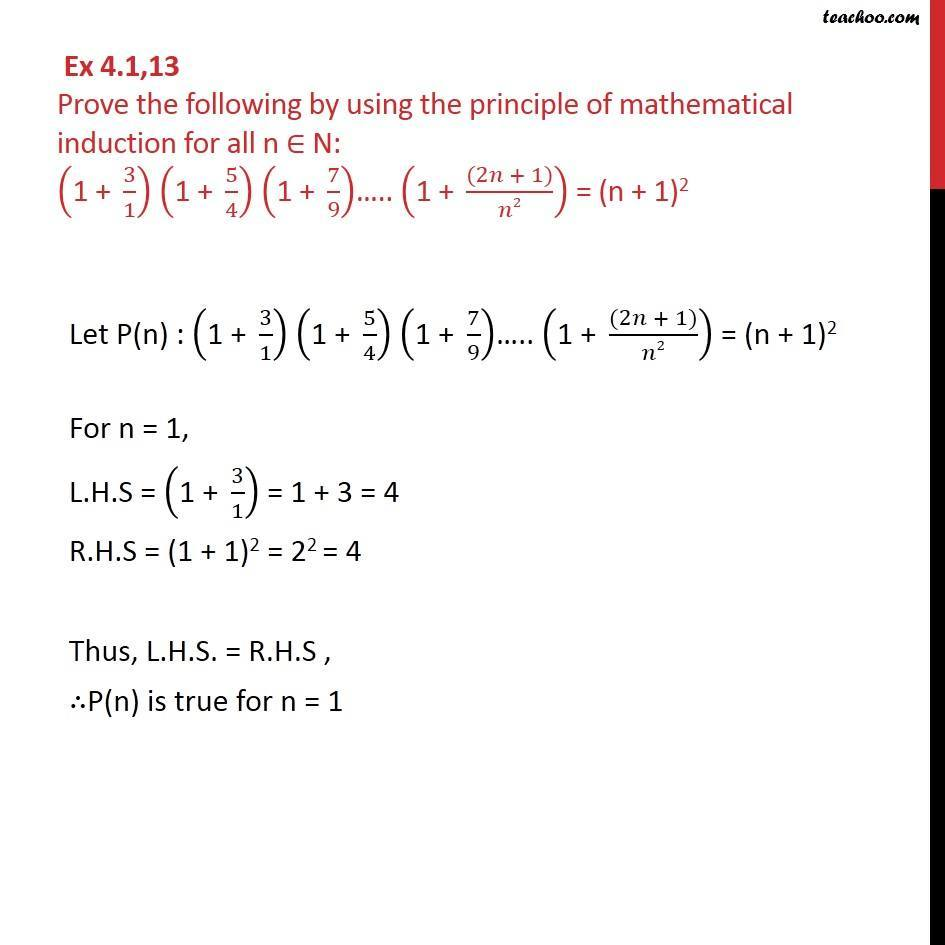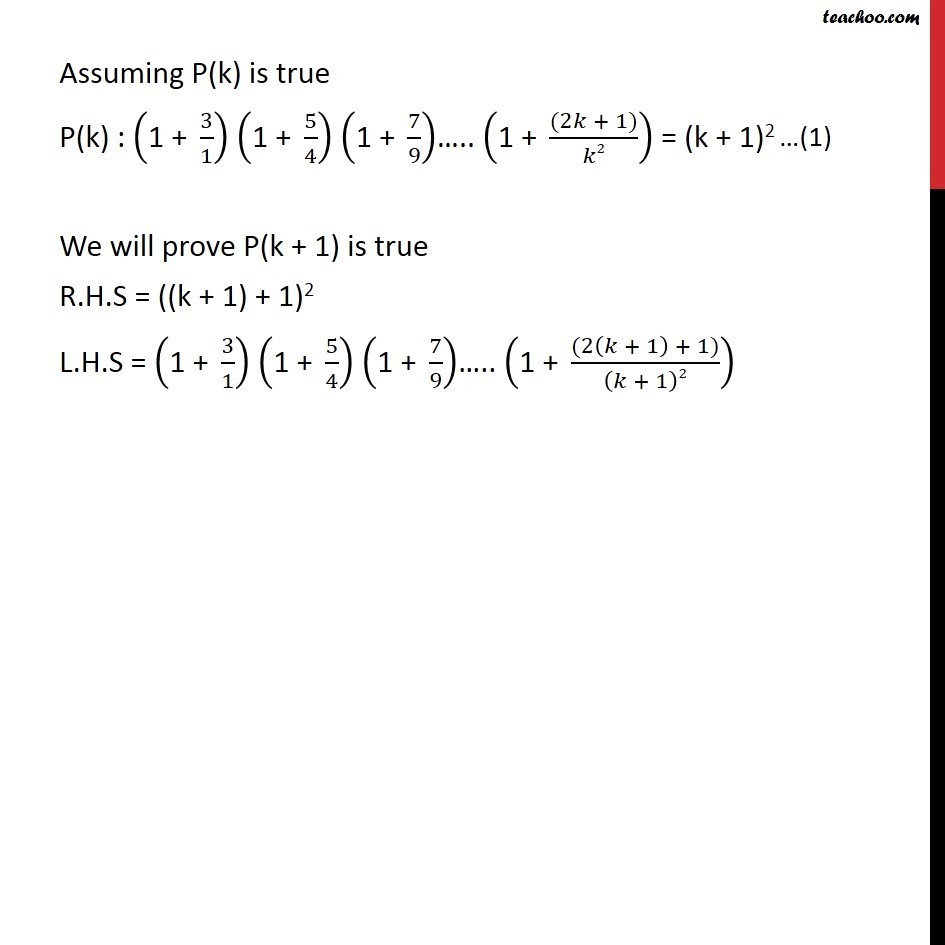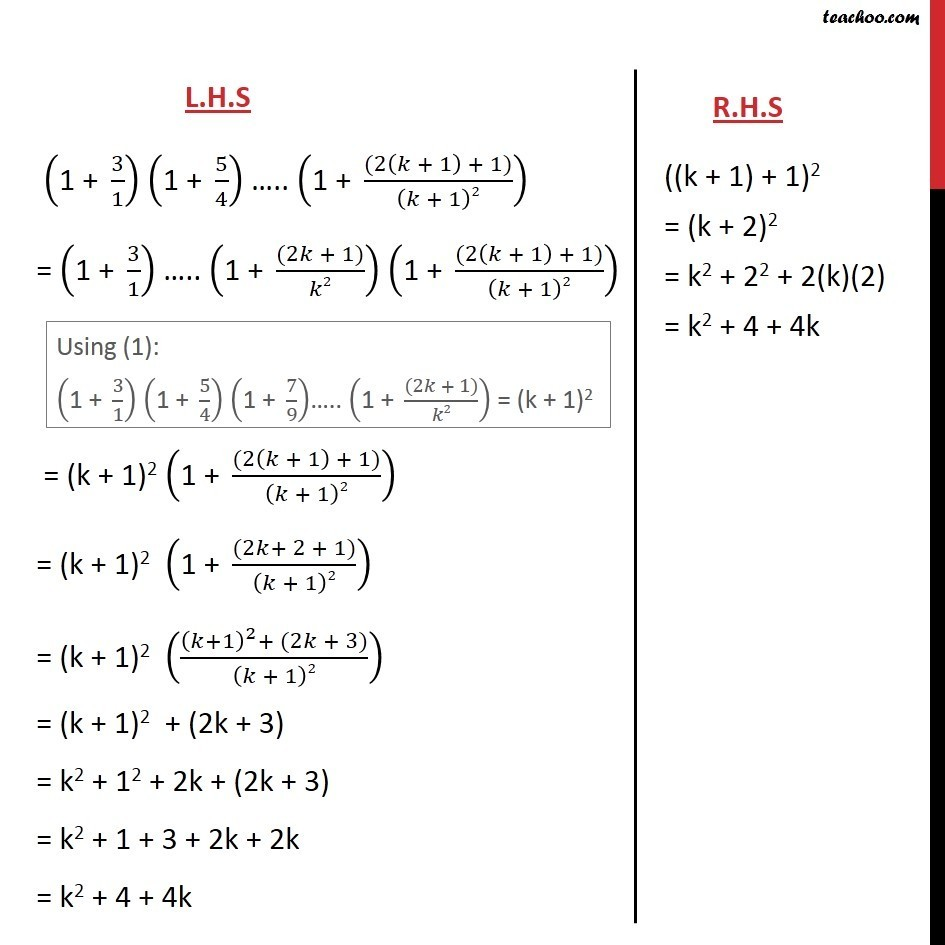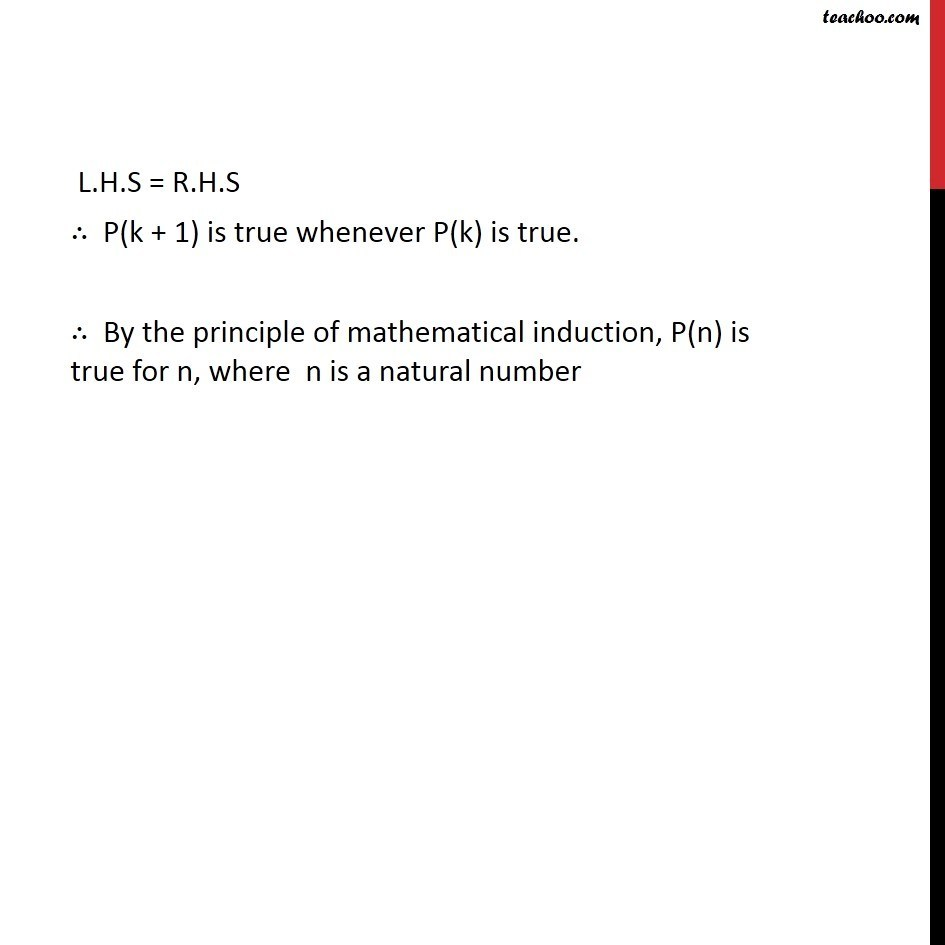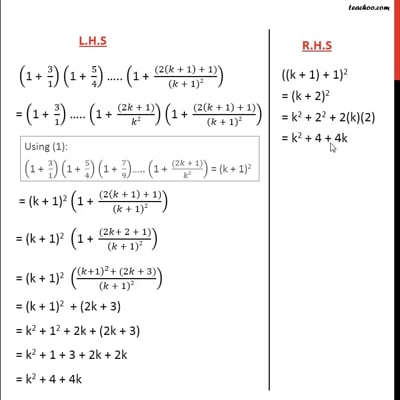This video is only available for Teachoo black users

Solve all your doubts with Teachoo Black (new monthly pack available now!)

### Transcript

Ex 4.1,13 Prove the following by using the principle of mathematical induction for all n ∈ N: ("1 + " 3/1) ("1 + " 5/4) ("1 + " 7/9)….. ("1 + " ((2𝑛 + 1))/𝑛2) = (n + 1)2 Let P(n) : ("1 + " 3/1) ("1 + " 5/4) ("1 + " 7/9)….. ("1 + " ((2𝑛 + 1))/𝑛2) = (n + 1)2 For n = 1, L.H.S = ("1 + " 3/1) = 1 + 3 = 4 R.H.S = (1 + 1)2 = 22 = 4 Thus, L.H.S. = R.H.S , ∴P(n) is true for n = 1 Assuming P(k) is true P(k) : ("1 + " 3/1) ("1 + " 5/4) ("1 + " 7/9)….. ("1 + " ((2𝑘 + 1))/𝑘2) = (k + 1)2 We will prove P(k + 1) is true R.H.S = ((k + 1) + 1)2 L.H.S = ("1 + " 3/1) ("1 + " 5/4) ("1 + " 7/9)….. ("1 + " ((2(𝑘 + 1) + 1))/(𝑘 + 1)2) L.H.S = R.H.S ∴ P(k + 1) is true whenever P(k) is true. ∴ By the principle of mathematical induction, P(n) is true for n, where n is a natural number